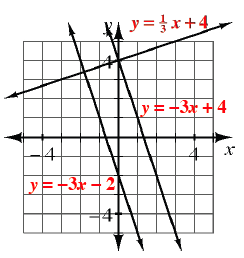### Home > GC > Chapter 1 > Lesson 1.2.5 > Problem1-92

1-92.

On graph paper, graph each of the lines below on the same set of axes. What is the relationship between lines (a) and (b)? What about between (b) and (c)?

1. $y =\frac{1}{3}x+4$

1. $y = −3x + 4$

1. $y = −3x − 2$